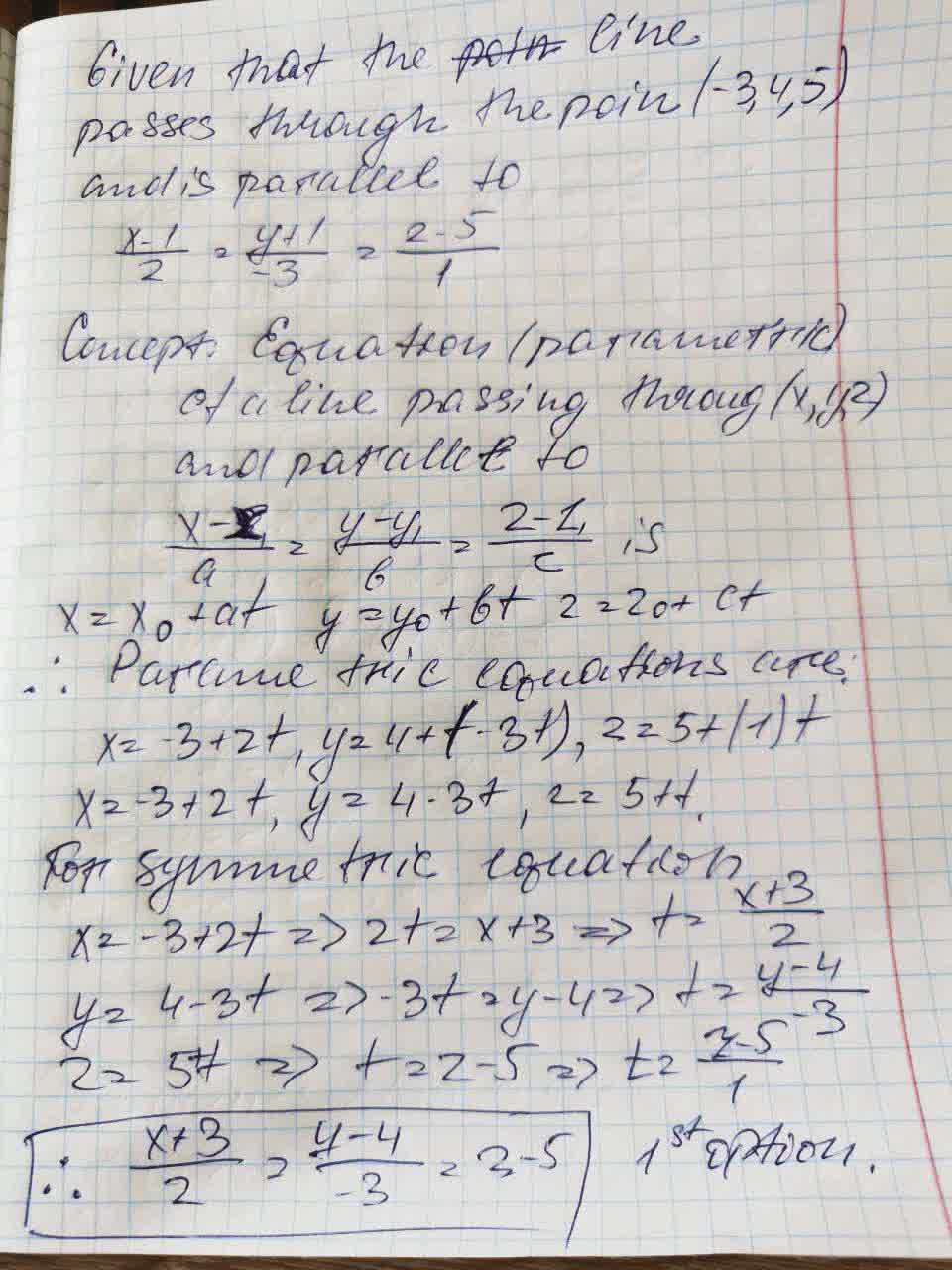# Find sets of parametric equations and symmetric equations of the line that passes through the given point and is parallel to the given vector or lineFalak Kinney 2021-09-15 Answered

Find sets of parametric equations and symmetric equations of the line that passes through the given point and is parallel to the given vector or line. (For each line, write the direction numbers as integers.)
Point: (-3, 4, 5)
Parallel to: $\frac{x-1}{2}=\frac{y+1}{-3}=z-5$
(a) Parametric equations
(b) Symmetric equations

You can still ask an expert for help

• Questions are typically answered in as fast as 30 minutes

Solve your problem for the price of one coffee

• Math expert for every subject
• Pay only if we can solve itMalena